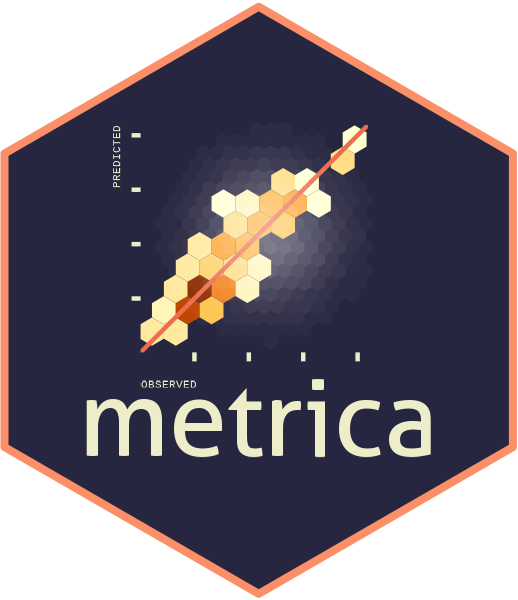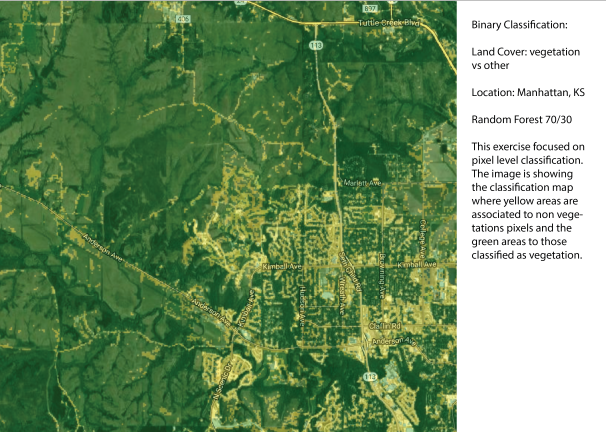# Classification case: Assessing the performance of remote sensing models

## 1. IntroductionThe `metrica` package was developed to visualize and compute the level of agreement between observed ground-truth values and model-derived (e.g., mechanistic or empirical) predicted.

This package is intended to fit into the following workflow:

1. a data set containing the observed values is used to train a model
2. the trained model is used to generate predicted
3. a data frame containing at least the observed and model-predicted values is created
4. `metrica` package is used to compute and evaluate the classification model based on observed and predicted values
5. `metrica` package is used to visualize model fit and selected fit metrics

This vignette introduces the functionality of the `metrica` package applied to observed and model-predicted values of a binary land cover classification scenario, where the two classes are vegetation (1) and non-vegetation (0)).

## Libraries

``````library(metrica)
library(dplyr)
library(purrr)
library(tidyr)``````

## 2. Example datasets

### 2.1. Kansas Land Cover dataNow we load the binary `land_cover` data set already included with the `metrica` package. This data set contains two columns:

• `predicted`: model-predicted (random forest) land cover, being vegetation = 1 and other = 0,

• `actual`: ground-truth observed land cover, being 0 = vegetation and 1 = other

``````# Load
binary_landCover <- metrica::land_cover

# Printing first observations